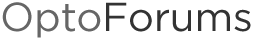# Weekly and monthly data tables

I have started to log my water use at home.
We installed a 1 pulse per gallon water meter, the output I have put to my EMU.
(Yeah, it shows up as Kw in the EMU Sensor Manger software, but I don’t use that, I use groov to look at the data, so I don’t care what it gets labeled in the EMU - as long as the scaling is right, 1 pulse per gallon sure sounds like 1 pulse per Kw).

Since the whole family looks at the data, I needed it to be a little friendly, so I have a day of the week table, and a month long table (31 days) and then a year long table (12 months).
This way we can keep track of day to day use, and see how we are going over the month.
I probably should bump this out to something like the last 5 years, so we can compare this years use with last years… If you cut the code for that, be sure and post it here! (Please).

Here is how I went about it…

``````
// Get the year/month/day in one transaction (so we avoid any problems w/values
// rolling over between individual reads)

// For the GetDateTime command: 0 = month, 1 = day of month, 2 = year,
// 3 = day of week, 4 = hours, 5 = minutes, 6 = seconds, 7 = milliseconds (unused)

// This command requires 9.1 or newer PAC Control and PAC firmware:
datetimestamp_status = GetDateTime(ntTimeTable);

// Year
NumberToString(ntTimeTable, sTemp);
while (GetStringLength(sTemp) < 4) sTemp = "0" + sTemp; wend // Pad with 0s
sDateTimestamp = sTemp;

// Month
NumberToString(ntTimeTable, sTemp);
while (GetStringLength(sTemp) < 2) sTemp = "0" + sTemp; wend // Pad with 0s
sDateTimestamp += "-";
sDateTimestamp += sTemp;

// Date
NumberToString(ntTimeTable, sTemp);
while (GetStringLength(sTemp) < 2) sTemp = "0" + sTemp; wend // Pad with 0s
sDateTimestamp += "-";
sDateTimestamp += sTemp;

sDateTimestamp += " "; // put a space after the date, before the time

// Hour
NumberToString(ntTimeTable, sTemp);
while (GetStringLength(sTemp) < 2) sTemp = "0" + sTemp; wend // Pad with 0s
sDateTimestamp += sTemp;

// Minute
NumberToString(ntTimeTable, sTemp);
while (GetStringLength(sTemp) < 2) sTemp = "0" + sTemp; wend // Pad with 0s
sDateTimestamp += ":";
sDateTimestamp += sTemp;

// Second
NumberToString(ntTimeTable, sTemp);
while (GetStringLength(sTemp) < 2) sTemp = "0" + sTemp; wend // Pad with 0s
sDateTimestamp += ":";
sDateTimestamp += sTemp;

seconds_since_midnight = GetSecondsSinceMidnight();

// get the current water count from the emu so we can see the use go up through the day.
water_daily_use = water_use_live_count - water_usage_daily_snapshot;

// get the daily water usage each night at midnight
if (seconds_since_midnight == 0) then
// get the current daily count and put it into the daily snapshot
water_usage_daily_snapshot = water_use_live_count;
water_weekly_use[ntTimeTable] = water_daily_use;

//yeah, its sorta a duplication from what we just did, one table is weekly, the other monthly.
// get the current daily snapshot and put it in the monthly table
water_monthly_use[ntTimeTable] = water_daily_use;

// Now check if we are at the start of the month. If so, add up the whole month and put the total in the yearly table.
if (ntTimeTable == 1) then
water_yearly_use[ntTimeTable] = 0;
for n1 = 1 to 31 step 1
water_yearly_use[ntTimeTable] = water_yearly_use[ntTimeTable]+ water_monthly_use[n1];
next
// Ok, now that we have added up the whole month, clear it. This way we don't have to keep track of 29 day years.
MoveToNumTableElements(0, 0, 31, water_monthly_use);
endif

// set the controller time once a day
time_zone_status = SetTimeZoneConfiguration("PST1,11,0,1,0200/PDT,3,0,2,0200");
time_sync_status = SynchronizeClockSNTP(5, time_server_url);

// Just do all the above once a day
delayMsec(1000);

endif

``````

Please note, I have only just done this code and its working over the past week, but I have not had a new month roll over yet.
So, if you see a bug, let me know quick!I put the code in place so we can start the new year logging data.

Enjoy.

Very nice!

You asked about storing data to compare year vs. year. Here’s how I would do it:

Go for 10 years, since we’re only talking < 500 more bytes and I’m guessing you have at least that much room for persistent variables. (For those not sure how much of each kind of memory you might have on your hardware, check out this handy tech note, form 1646.)

Add a new table for your 10-year data (keep the existing table to use in your HMI/groov), let’s say it’s called: [B]water_decade_use[/B] – a persistent float table with 121 elements in it (the extra element is because we’ll start at month 1–what’s returned from your GetDateTime–rather than 0, where the table starts).

You [I]could [/I]add just one line of code, after your for loop where you calculate your [B]water_yearly_use[/B] values. It would look something like this:[INDENT]

``````water_decade_use[ ((2013 - ntTimeTable) % 10) + ntTimeTable ]  = water_yearly_use[ntTimeTable];
``````

[/INDENT]But I’d recommend adding a few more variables, just for readability, especially since you might not remember which elements in that ntTimeTable our GetDateTime returns off-hand, and that calculation might give you a headache anyway. You could create this constants:[INDENT][B]MONTH_INDEX = 0;
DAY_OF_MONTH_INDEX = 1;
YEAR_INDEX = 2; [/B]

[/INDENT]And one more extra variable to calculate the index in one extra step, something like this:[INDENT]

``````decade_table_index = ((2013 - ntTimeTable[YEAR_INDEX]) % 10) + ntTimeTable[MONTH_INDEX];